Next: Exercises Up: Orbital Angular Momentum Previous: Motion in Central Field

Energy Levels of Hydrogen Atom

Consider a hydrogen atom, for which the potential takes the specific form(4.119)

The radial eigenfunction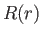satisfies Equation (4.118), which can be written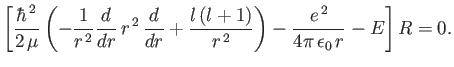(4.120)

Here,is the reduced mass, which takes into account the fact that the electron (of mass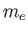) and the proton (of mass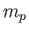) both orbit about a common centre of mass, which is equivalent to a particle of massorbiting about a fixed point . However, given that, we can safely replaceby. (The correction involved in using, rather than, in the analysis is actually less than that involved in neglecting the electron's relativistic mass increase.) Let us write the product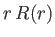as the function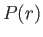. The previous equation transforms to(4.121)

which is the one-dimensional Schrödinger equation for a particle of massmoving in the effective potential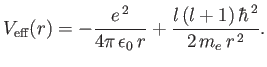(4.122)

The effective potential has a simple physical interpretation. The first part is the attractive Coulomb potential, and the second part corresponds to the repulsive centrifugal force.

Let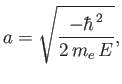(4.123)

and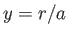, with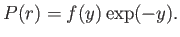(4.124)

Here, it is assumed that the energy eigenvalueis negative. Equation (4.121) transforms to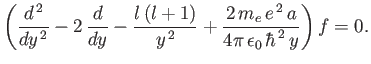(4.125)

Let us look for a power-law solution of the form(4.126)

Substituting this solution into Equation (4.125), we obtain(4.127)

Equating the coefficients ofgives(4.128)

Now, the power-law series (4.126) must terminate at small, at some positive value of, otherwise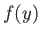would diverge unphysically as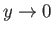. This is only possible if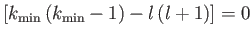, where the first term in the series is. There are two possibilities:or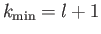. The former predicts unphysical divergence of the wavefunction at. Thus, we conclude that, and that the first term in the series is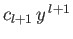. Note that for an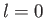state there is a finite probability of finding the electron at the nucleus, whereas for anstate there is zero probability of finding the electron at the nucleus (i.e.,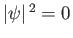at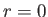, except when). Furthermore, it is only possible to obtain sensible behavior of the wavefunction as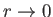if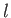is an integer.

For large values of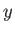, the ratio of successive terms in the series (4.126) is(4.129)

according to Equation (4.128). This is the same as the ratio of successive terms in the series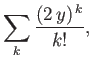(4.130)

which converges to. We conclude thatas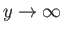. It follows from Equation (4.124) that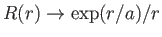as. This does not correspond to physically acceptable behavior of the wavefunction, becausemust be finite. The only way in which we can avoid this unphysical behavior is if the series (4.126) terminates at some maximum value of. According to the recursion relation (4.128), this is only possible if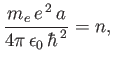(4.131)

where the last term in the series is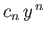. It follows from Equation (4.123) that the energy eigenvalues are quantized, and can only take the values(4.132)

where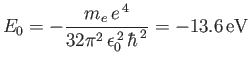(4.133)

is the energy of the ground state (i.e., the lowest energy state). Here,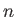is a positive integer that must exceed the quantum number, otherwise there would be no terms in the series (4.126). Expression (4.132) is known as the Bohr formula, because it was derived heuristically by Niels Bohr in 1913 .

In summary, the properly normalized wavefunction of a hydrogen atom is written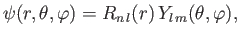(4.134)

where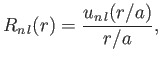(4.135)

and(4.136)

Here,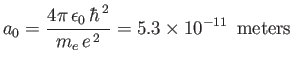(4.137)

is the Bohr radius, and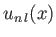is a well-behaved solution of the differential equation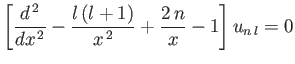(4.138)

that satisfies the normalization constraint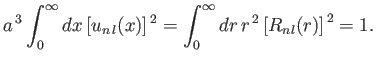(4.139)

Finally, the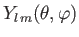are spherical harmonics. The restrictions on the quantum numbers are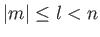, whereis a positive integer,a non-negative integer, and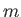an integer. Incidentally, the quantum numbers,, andare conventionally referred to as the principle quantum number, the azimuthal quantum number, and the magnetic quantum number, respectively.

The ground state of hydrogen corresponds to. The only permissible values of the other quantum numbers areand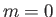. Thus, the ground state is a spherically symmetric, zero angular momentum, state. The next energy level corresponds to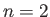. The other quantum numbers are allowed to take the values,or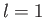,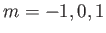. Thus, there arestates with non-zero angular momentum. Note that the energy levels given in Equation (4.132) are independent of the quantum number, despite the fact thatappears in the radial eigenfunction equation (4.138). This is a special property of a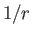Coulomb potential.

In addition to the quantized negative energy states of the hydrogen atom, which we have just found, there is also a continuum of unbound positive energy states.Next: Exercises Up: Orbital Angular Momentum Previous: Motion in Central Field
Richard Fitzpatrick 2016-01-22